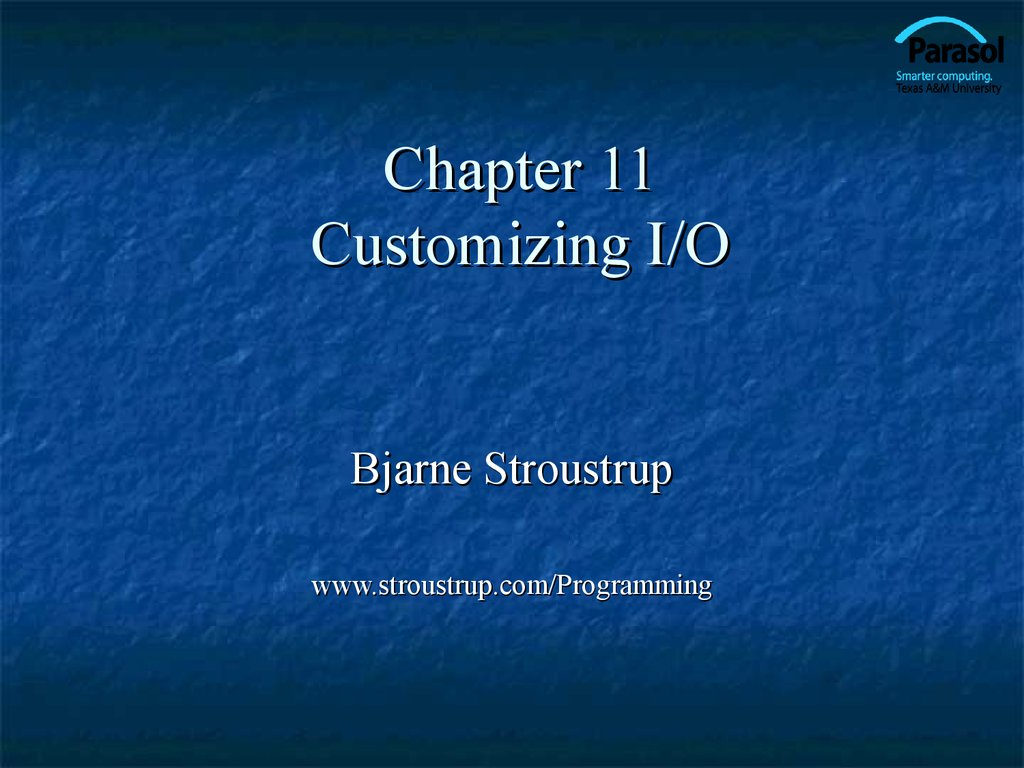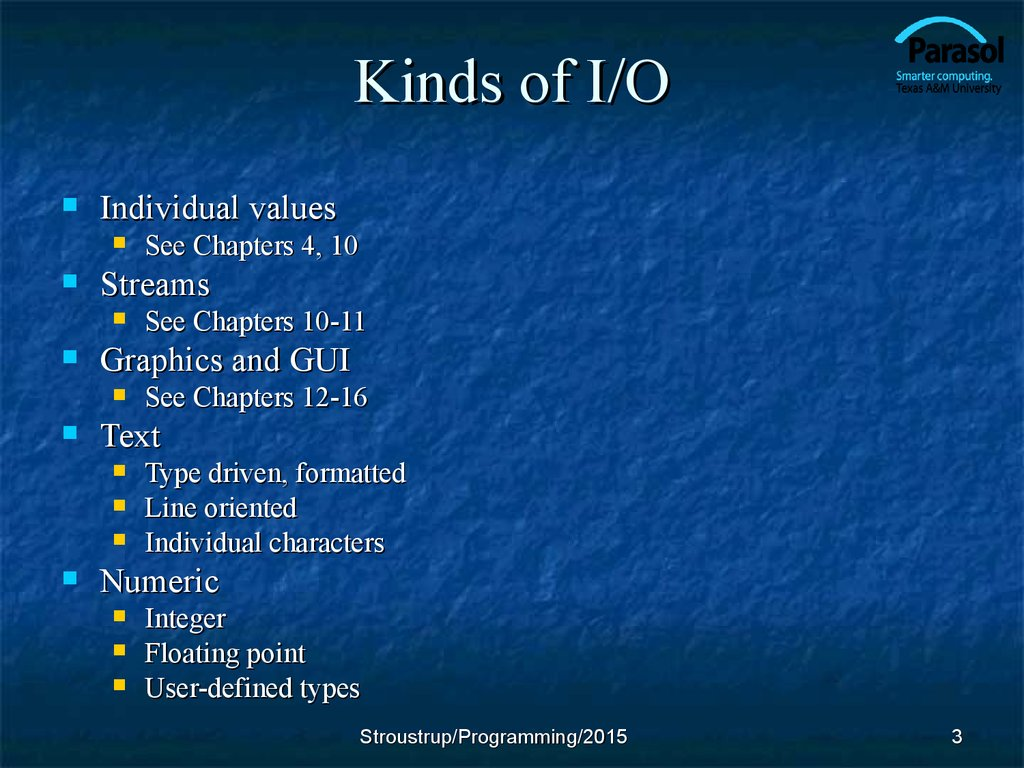# Customizing I/O. (Chapter 11)

## 1. Chapter 11 Customizing I/O

Bjarne Stroustrup
www.stroustrup.com/Programming

## 2. Overview

Input and output
Numeric output
File modes
Integer
Floating point
Binary I/O
Positioning
String streams
Line-oriented input
Character input
Character classification
Stroustrup/Programming/2015
2

## 3. Kinds of I/O

Individual values
Streams
See Chapters 12-16
Text
See Chapters 10-11
Graphics and GUI
See Chapters 4, 10
Type driven, formatted
Line oriented
Individual characters
Numeric
Integer
Floating point
User-defined types
Stroustrup/Programming/2015
3

## 4. Observation

As programmers we prefer regularity and simplicity
But, our job is to meet people’s expectations
People are very fussy/particular/picky about the way
their output looks
They often have good reasons to be
What does 110 mean?
What does 123,456 mean?
What does (123) mean?
The world (of output formats) is weirder than you could
possibly imagine
Stroustrup/Programming/2015
4

## 5. Output formats

Integer values
1234.57
1.2345678e+03
1234.567890
(general)
(scientific)
(fixed)
Precision (for floating-point values)
(decimal)
(octal)
Floating point values
1234
2322
4d2
1234.57
1234.6
(precision 6)
(precision 5)
Fields
|12|
| 12|
(default for | followed by 12 followed by |)
(12 in a field of 4 characters)
Stroustrup/Programming/2015
5

## 6. Numerical Base Output

You can change “base”
Base 10 == decimal; digits: 0 1 2 3 4 5 6 7 8 9
Base 8 == octal; digits: 0 1 2 3 4 5 6 7
Base 16 == hexadecimal; digits: 0 1 2 3 4 5 6 7 8 9 a b c d e f
// simple test:
cout << dec << 1234 << "\t(decimal)\n"
<< hex << 1234 << "\t(hexadecimal)\n"
<< oct << 1234 << "\t(octal)\n";
// The '\t' character is “tab” (short for “tabulation character”)
// results:
1234
4d2
2322
(decimal)
(octal)
Stroustrup/Programming/2015
6

## 7. “Sticky” Manipulators

You can change “base”
Base 10 == decimal; digits: 0 1 2 3 4 5 6 7 8 9
Base 8 == octal; digits: 0 1 2 3 4 5 6 7
Base 16 == hexadecimal; digits: 0 1 2 3 4 5 6 7 8 9 a b c d e f
// simple test:
cout << 1234 << '\t'
<< hex << 1234 << '\t'
<< oct << 1234 << '\n';
cout << 1234 << '\n';
// the octal base is still in effect
// results:
1234
2322
4d2
2322
Stroustrup/Programming/2015
7

## 8. Other Manipulators

You can change “base”
Base 10 == decimal; digits: 0 1 2 3 4 5 6 7 8 9
Base 8 == octal; digits: 0 1 2 3 4 5 6 7
Base 16 == hexadecimal; digits: 0 1 2 3 4 5 6 7 8 9 a b c d e f
// simple test:
cout << 1234 << '\t'
<< hex << 1234 << '\t'
<< oct << 1234 << endl;
cout << showbase << dec; // show bases
cout << 1234 << '\t'
<< hex << 1234 << '\t'
<< oct << 1234 << '\n';
// results:
1234
4d2
2322
1234
0x4d2 02322
Stroustrup/Programming/2015
// '\n'
8

## 9. Floating-point Manipulators

You can change floating-point output format
defaultfloat – iostream chooses best format using n digits (this is the default)
scientific – one digit before the decimal point plus exponent; n digits after .
fixed – no exponent; n digits after the decimal point
// simple test:
cout << 1234.56789 << "\t\t(defaultfloat)\n" // \t\t to line up columns
<< fixed << 1234.56789 << "\t(fixed)\n"
<< scientific << 1234.56789 << "\t(scientific)\n";
// results:
1234.57
1234.567890
1.234568e+03
(defaultfloat)
(fixed)
(scientific)
Stroustrup/Programming/2015
9

## 10. Precision Manipulator

Precision (the default is 6)
defaultfloat – precision is the number of digits
scientific – precision is the number of digits after the . (dot)
fixed – precision is the number of digits after the . (dot)
// example:
cout << 1234.56789 << '\t' << fixed << 1234.56789 << '\t'
<< scientific << 1234.56789 << '\n';
cout << general << setprecision(5)
<< 1234.56789 << '\t' << fixed << 1234.56789 << '\t'
<< scientific << 1234.56789 << '\n';
cout << general << setprecision(8)
<< 1234.56789 << '\t' << fixed << 1234.56789 << '\t'
<< scientific << 1234.56789 << '\n';
// results (note the rounding):
1234.57
1234.567890
1.234568e+03
1234.6
1234.56789
1.23457e+03
1234.5679
1234.56789000
1.23456789e+03
Stroustrup/Programming/2015
10

## 11. Output field width

A width is the number of characters to be used for the next
output operation
Beware: width applies to next output only (it doesn’t “stick” like
precision, base, and floating-point format)
Beware: output is never truncated to fit into field
// example:
cout << 123456 <<'|'<< setw(4) << 123456 << '|'
<< setw(8) << 123456 << '|' << 123456 << "|\n";
cout << 1234.56 <<'|'<< setw(4) << 1234.56 << '|'
<< setw(8) << 1234.56 << '|' << 1234.56 << "|\n";
cout << "asdfgh" <<'|'<< setw(4) << "asdfgh" << '|'
<< setw(8) << "asdfgh" << '|' << "asdfgh" << "|\n";
// results:
123456|123456| 123456|123456|
1234.56|1234.56| 1234.56|1234.56|
asdfgh|asdfgh| asdfgh|asdfgh|
Stroustrup/Programming/2015
11

## 12. Observation

This kind of detail is what you need textbooks,
manuals, references, online support, etc. for
You always forget some of the details when you need them
Stroustrup/Programming/2015
12

## 13. A file

0:
1:
2:
At the fundamental level, a file is a sequence of
bytes numbered from 0 upwards
Other notions can be supplied by programs that
interpret a “file format”
For example, the 6 bytes "123.45" might be interpreted
as the floating-point number 123.45
Stroustrup/Programming/2015
13

## 14. File open modes

By default, an ifstream opens its file for reading
By default, an ofstream opens its file for writing.
Alternatives:
ios_base::app
// append (i.e., output adds to the end of the file)
ios_base::ate
// “at end” (open and seek to end)
ios_base::binary // binary mode – beware of system specific behavior
ios_base::in
ios_base::out
// for writing
ios_base::trunc // truncate file to 0-length
A file mode is optionally specified after the name of the file:
ofstream of1 {name1}; // defaults to ios_base::out
ifstream if1 {name2}; // defaults to ios_base::in
ofstream ofs {name, ios_base::app}; // append rather than overwrite
fstream fs {"myfile", ios_base::in|ios_base::out}; // both in and out
Stroustrup/Programming/2015
14

## 15. Text vs. binary files

123 as characters:
1
2
3
?
?
?
?
?
12345 as characters:
1
2
3
4
5
?
?
?
123 as binary:
00000000
01111011
12345 as binary:
00110000
00111001
123456 as characters:
1
2
3
123 456 as
characters:
1
2
3
In binary files, we use
sizes to delimit values
4
5
6
4
5
?
In text files, we use
separation/termination
characters
6
Stroustrup/Programming/2015
15

## 16. Text vs. binary

Use text when you can
You can read it (without a fancy program)
You can debug your programs more easily
Text is portable across different systems
Most information can be represented reasonably as text
Use binary when you must
E.g. image files, sound files
Stroustrup/Programming/2015
16

## 17. Binary files

int main()
// use binary input and output
{
cout << "Please enter input file name\n";
string iname;
cin >> iname;
ifstream ifs {iname,ios_base::binary}; // note: binary
if (!ifs) error("can't open input file ", iname);
cout << "Please enter output file name\n";
string oname;
cin >> oname;
ofstream ofs {oname,ios_base::binary};
// note: binary
if (!ofs) error("can't open output file ", oname);
// “binary” tells the stream not to try anything clever with the bytes
Stroustrup/Programming/2015
17

## 18. Binary files

vector<int> v;
v.push_back(i);
// … do something with v …
// write to binary file:
for(int i=0; i<v.size(); ++i)
ofs.write(as_bytes(v[i]),sizeof(int));
return 0;
// note: writing bytes
}
// For now, treat as_bytes() as a primitive
// Warning! Beware transferring between different systems
Stroustrup/Programming/2015
18

## 19. Positioning in a filestream

Put position: 2
0:
A file:
Get position: 6
1:
y
2:
3:
4:
5:
6:
x

fstream fs {name};
// open for input and output
// …
fs.seekg(5);
// move reading position (‘g’ for ‘get’) to 5 (the 6th character)
char ch;
fs>>ch;
cout << "sixth character is " << ch << '(' << int(ch) << ")\n";
fs.seekp(1);
// move writing position (‘p’ for ‘put’) to 1 (the 2nd character)
fs<<'y';
// write and increment writing position to 2
Stroustrup/Programming/2015
19

## 20. Positioning

Whenever you can
Use simple streaming
Streams/streaming is a very powerful metaphor
Write most of your code in terms of “plain” istream and ostream
Positioning is far more error-prone
Handling of the end of file position is system dependent and
basically unchecked
Stroustrup/Programming/2015
20

## 21. String streams

A stringstream reads/writes from/to a string
rather than a file or a keyboard/screen
double str_to_double(string s)
// if possible, convert characters in s to floating-point value
{
istringstream is {s}; // make a stream so that we can read from s
double d;
is >> d;
if (!is) error("double format error: “,s);
return d;
}
double d1 = str_to_double("12.4");
// testing
double d2 = str_to_double("1.34e-3");
double d3 = str_to_double("twelve point three"); // will call error()
Stroustrup/Programming/2015
21

## 22. String streams

See textbook for ostringstream
String streams are very useful for
formatting into a fixed-sized space (think GUI)
for extracting typed objects out of a string
Stroustrup/Programming/2015
22

## 23. Type vs. line

string name;
cin >> name;
cout << name << '\n';
// input: Dennis Ritchie
// output: Dennis
string name;
getline(cin,name);
// input: Dennis Ritchie
cout << name << '\n';
// output: Dennis Ritchie
// now what?
// maybe:
istringstream ss(name);
ss>>first_name;
ss>>second_name;
Stroustrup/Programming/2015
23

## 24. Characters

You can also read individual characters
for (char ch; cin>>ch; ) {
// read into ch, skip whitespace characters
if (isalpha(ch)) {
// do something
}
}
for (char ch; cin.get(ch); ) { // read into ch, don’t skip whitespace characters
if (isspace(ch)) {
// do something
}
else if (isalpha(ch)) {
// do something else
}
}
Stroustrup/Programming/2015
24

## 25. Character classification functions

If you use character input, you often need one or
more of these (from header <cctype> ):
isspace(c)
isalpha(c)
isdigit(c)
isupper(c)
islower(c)
isalnum(c)
// is c whitespace? (' ', '\t', '\n', etc.)
// is c a letter? ('a'..'z', 'A'..'Z') note: not '_'
// is c a decimal digit? ('0'..'9')
// is c an upper case letter?
// is c a lower case letter?
// is c a letter or a decimal digit?
etc.
Stroustrup/Programming/2015
25

## 26. Line-oriented input

Prefer >> to getline()
i.e. avoid line-oriented input when you can
People often use getline() because they see no alternative
But it easily gets messy
When trying to use getline(), you often end up
using >> to parse the line from a stringstream
using get() to read individual characters
Stroustrup/Programming/2015
26

## 27. C++14

Binary literals
Digit separators
0b1010100100000011
0b1010'1001'0000'0011
Can also be used for for decimal, octal, and hexadecimal numbers
User-Defined Literals (UDLs) in the standard library
Time: 2h+10m+12s+123ms+3456ns
Complex: 2+4i
Stroustrup/Programming/2015
27

## 28. Next lecture

Graphical output
Creating a window
Drawing graphs
Stroustrup/Programming/2015
28